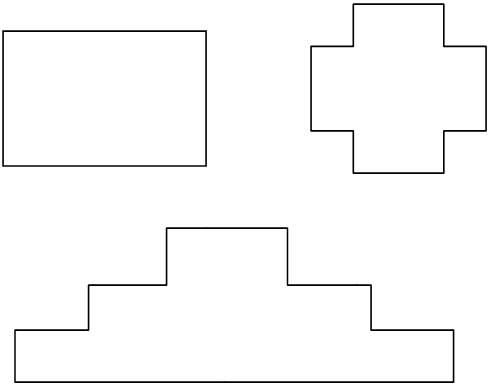Courses
Courses for Kids
Free study material
Offline Centres
MoreLast updated date: 04th Dec 2023
Total views: 381.9k
Views today: 10.81k

# Rectilinear figure is formed by:A. Plane B. PointsC. Straight Lines D. Can’t be determinedVerified
Hint- In geometry, a rectilinear figure can be defined as a plane figure or shape whose sides meet at right angles. These edges are formed by straight lines so as to form a polygon with all interior angles of ${90^0}.$The interior angle at each vertex of the rectilinear is ${90^0}$ or ${270^0}$ . Here, for instance, a few irregular polygons that classify as rectilinear figures.
Note- A plane figure whose boundaries are line segments is called a rectilinear figure.Students must not confuse a rectilinear figure with a polygon. A rectilinear figure may be closed or open. Also all the interior angles of a rectilinear figure are the same and equal to ${90^0}$ or ${270^0}$.